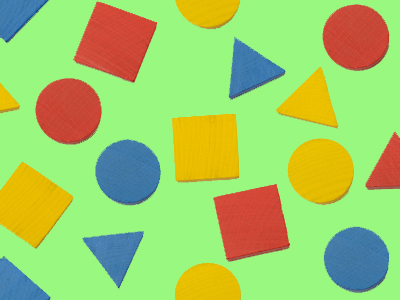Triangles, circles and squares are three of the most common 2-dimensional shapes.

# Year 1 Shapes - 2D

This quiz addresses the requirements of the National Curriculum KS1 in Maths and Numeracy for children aged 5 and 6 in year 1. Specifically this quiz is aimed at the section dealing with recognising and naming common 2-D shapes.

Year 1 children will learn to recognise and name basic and common 2-D shapes such as squares, circles and triangles. They will use 2-dimensional shapes to make repeating patterns and, as these shapes are common to the real world, the children will be encouraged to spot and recognise them and also to correctly name them.

To see a larger image, click on the picture.
Question 1
What shape is the clock?
A circle
A triangle
An oblong
A square
It has four equal sides
Question 2
This is a pattern made of...
Circles
Oblongs
Squares
Triangles
There are rows and rows of squares in the pattern
Question 3
These people are standing in a...
Triangle
Square
Circle
Oblong
They are making a round shape - a circle
Question 4
These bricks are all..
Circles
Triangles
Squares
Oblongs
The oblongs are making a herringbone pattern
Question 5
The board is a...
Rectangle
Square
Triangle
Circle
The board is rectangular and also an oblong
Question 6
What shape makes up this pattern?
Square
Triangle
Oblong
Circle
They are large and small triangles
Question 7
What shape is this person making with their hands?
Triangle
Square
Circle
Oblong
They are making the three sides of a triangle
Question 8
A square could also be called a...
Triangle
Circle
Oblong
Rectangle
Rectangles must have 4 sides, four 90 degree angles and two pairs of parallel sides
Question 9
This pattern is made up of...
Squares
Circles
Oblongs
Triangles
There are rows of squares in this pattern
Question 10
What shape is around these snooker balls?
A circle
A square
A triangle
An oblong
It's a triangular frame